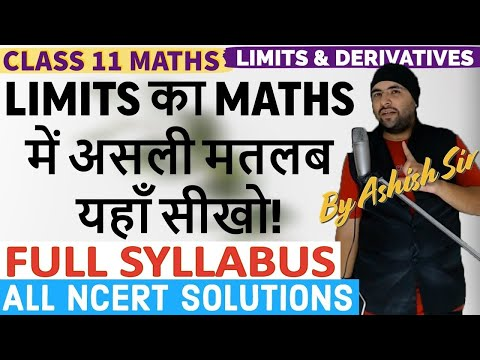Ashish Kumar - Let's Learn► Play all

Chapter 13 Limits And Derivatives Class 11 Maths IIT JEE Mains

Complete Syllabus of Class 11 Maths Chapter 13 Limits And Derivatives:
Derivative introduced as rate of change both as that of distance function and geometrically.Intuitive idea of limit.Limits of polynomials and rational functions trigonometric, exponential and logarithmic functions.Definition of derivative relate it to scope of tangent of the curve, derivative of sum, difference, product and quotient of functions. Derivatives of polynomial and trigonometric functions.
NCERT Exercise 13.1
NCERT Exercise 13.2
NCERT Supplementary Exercise
NCERT Miscellaneous Exercise
NCERT Chapter 13 Examples

Main Website:
https://www.ashishkumarletslearn.com

#LimitsAndDerivatives #Class11Maths
Complete Syllabus of Class 11 Maths Chapter 13 Limits And Derivatives:
Derivative introduced as rate of change both as that of distance function and geometrically.Intuitive idea of limit.Limits of polynomials and rational functions trigonometric, ...